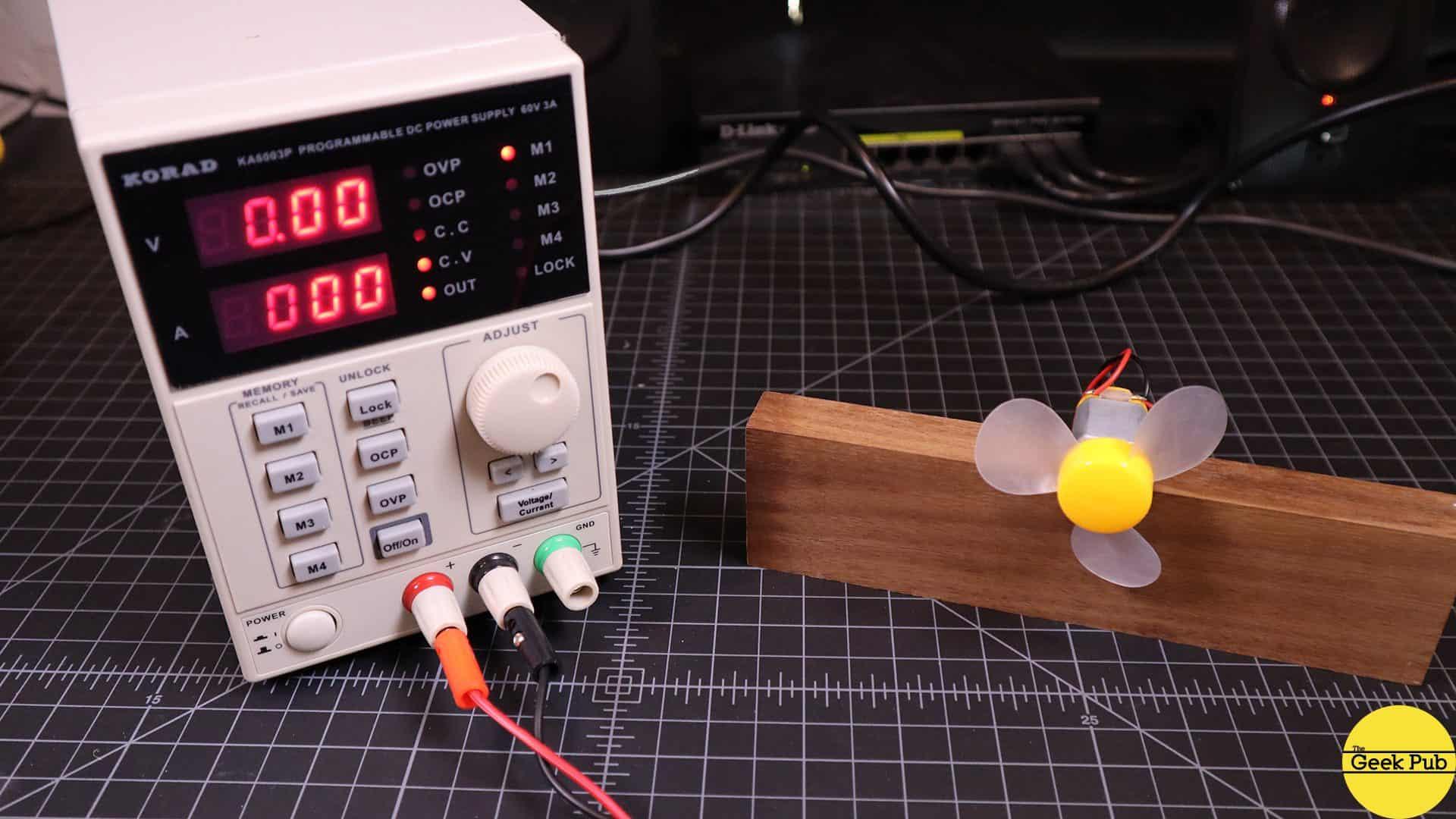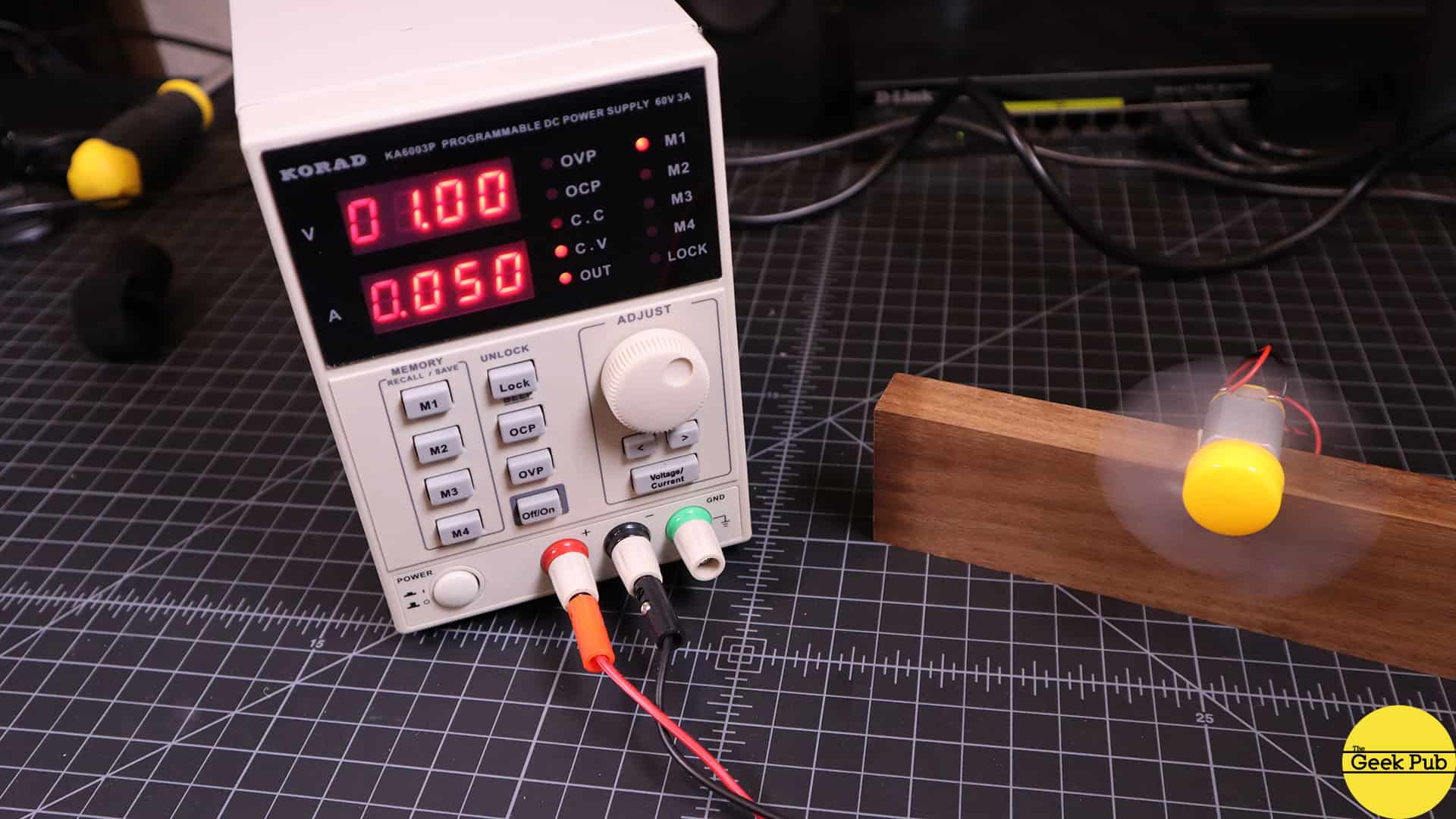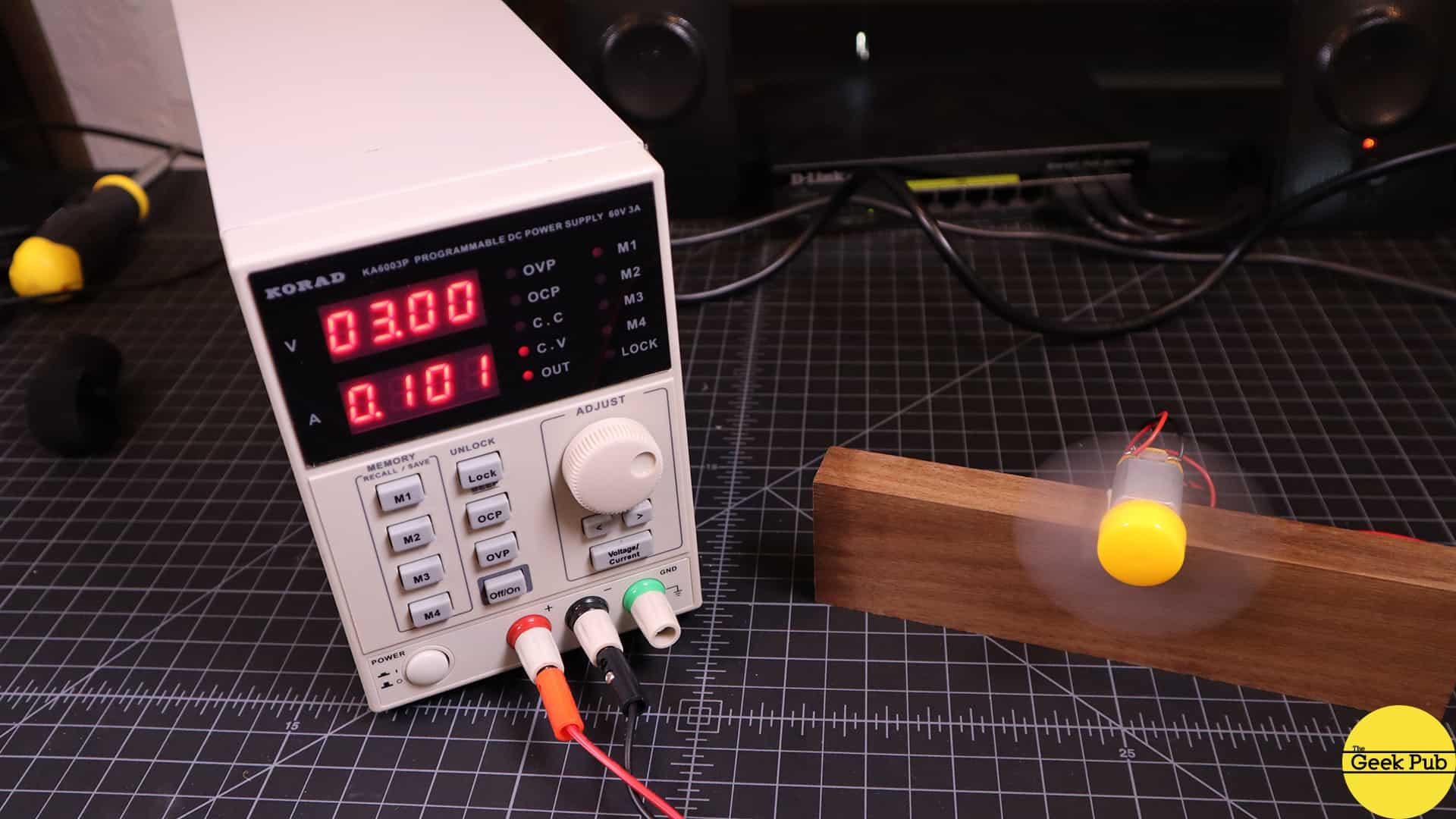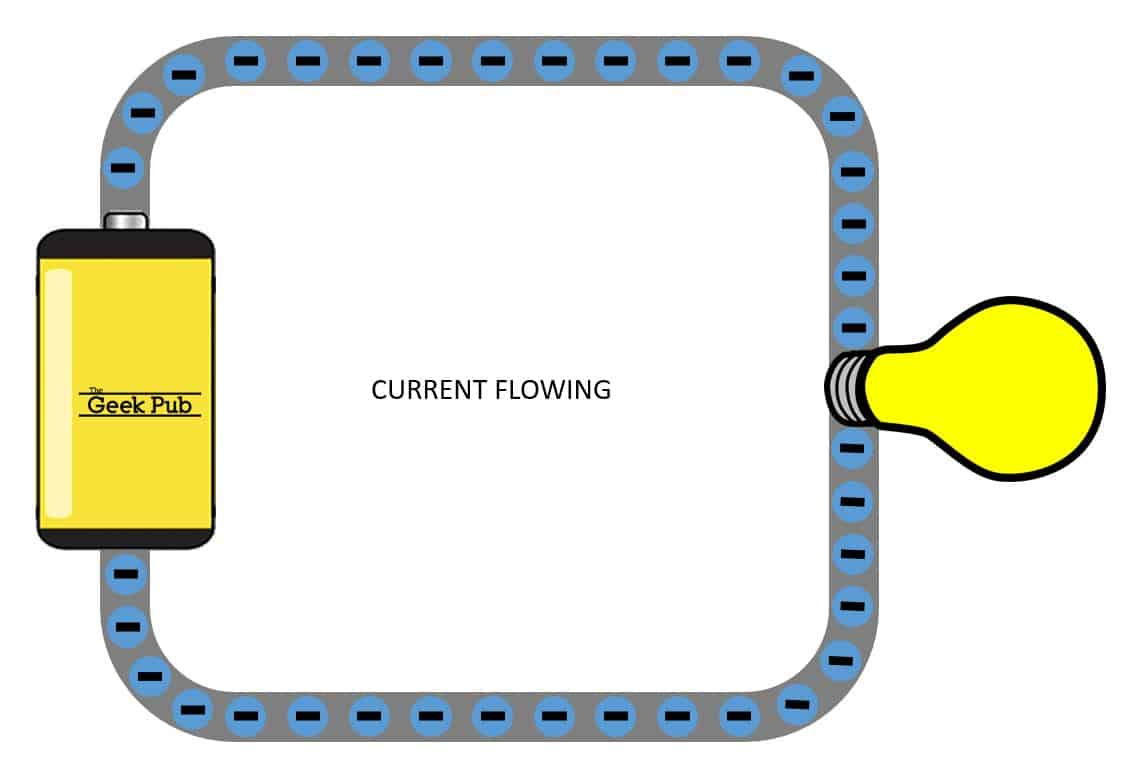In our first tutorial about basic electricity, you learned that current is just the flow of electrons in a wire. You learned about current and that its unit of measurement Amps refers to how many electrons are flowing past a certain point per second. In this tutorial we’re going to answer the questions you’ve all been asking: “What is voltage?”!  So what is voltage and what does it actually do?  Let’s find out!

## What is Voltage?

Simply explained, voltage pushes current around an electric circuit. Think of voltage like a pushing force, forcing electrons to start moving around.  This creates an electric current. Let’s look at an example. In the picture below I have an adjustable power supply connected to a small motor.

As we increase the voltage going to the motor, more current flows. When we have the power supply set to zero volts nothing happens, and the motor does not turn.If we set the power supply to output 1 volt. Immediately you can see that there is current flowing roughly 50 milliamps. When there’s current flowing, energy can flow from the power supply to the motor!OK! So now let’s increase the voltage to two volts! Now that we have bigger pushing force, more current is flowing.  It reaches just over 100 milliamps. You can see that more energy is flowing through the motor and it is turning much faster. The more we increase the voltage, we get even more current and the motor speeds up.You can use a voltage source to power motors, light bulbs, electronics, and many other things.

## Voltage Can Transmit Information

Another important example of what voltage can do is carry useful information, or data!

You can use different voltages as electrical signals that represent data. In this example, 0 volts or 5 volts represents binary 0s and 1s respectively in a communications bus. We will have a more advanced tutorial on this topic in the future, but I just want you to understand that sometimes voltage is used to power things that draw high amounts of current, but you can also use voltage as a signal, and almost no current flows at all. You just create the changing voltages with a transmitter, and detect the changing voltages with a suitable receiver.

You can see an example of voltage used to transmit data here on the oscilloscope.  Zero volts, followed by 5 volt pulses create a signal that can be read by another device.

RELATED: How Oscilloscopes Work## Do Volts Really Push Amps?  Sort of.

Now we’ve simply described it is “volts push amps”. Let’s dive a little deeper! There’s a chemical reaction inside these Geek Pub batteries that’s creating a voltage. The bottom side of the battery is more negatively charged than the top of the battery. Remember from our current tutorial that negative charges repel negative charges, so the bottom side of the battery will push electrons away from it.

Electrons push other electrons. Current flows and the electrons on the top side get attracted to the positive side of the battery, and everything flows in a complete circuit.## Understanding Voltage

Now let’s dive a little deeper and talk about what voltage actually is. Voltage is a difference in electrical potential energy, per unit of charge between two points.

Let’s break this down a bit more.  It’s super handy to understand these terms.

### Potential Energy

Let’s start with the potential energy. The scientific definition of energy is the ability to do work. This work could be moving something, heating something, turning something, or the like. Something has potential energy if it has the potential to do work. For example, a stretched elastic band has elastic potential energy. It’s not doing anything right now, but it has the potential to do work. If you release it, the elastic potential energy would be converted into movement that propels the piece of paper into your target.Back to our Geek Pub batteries above. They have electrical potential energy. It’s not doing anything when not connected, but it does have the potential to do work. There’s a chemical reaction inside it that creates electrical potential energy and if we connect a light to the battery we form a complete electrical circuit. Current will flows and the bulb lights up.

## Joules and Coulombs Explained

We’re almost done answering our question of “What is voltage?” But it’s also important to know that we measure energy with units called joules. Joules can be used to describe the amount of energy it takes to do a lot of different things. 1 joule is enough energy to power a flashlight for about 1 second. 3 joules is enough energy to power a flashlight for about 3 seconds. 90,000 joules is the energy required to power a microwave for 1 minute to make a cup of hot tea.

Now that you have an idea of what electrical potential energy means, what does this unit of charge mean? If you remember our tutorial on current, we said  that electrons are negatively charged particles, and 1 amp is 6.24 x 10^18 electrons flowing per second. That’s a really hard to remember number, and engineers don’t really ever use it. Instead, they use a standard unit of charge.  It’s called the coulomb.

The total charge on 6.24 x 10^18 electrons is equal to 1 coulomb of charge. And since electrons have a negative charge, this charge would be negative. It’s much easier to just say 1 ampere is equal to 1 coulomb of charge flowing per second. And 2 amps is 2 coulombs flowing per second.

When we talk about electrical potential energy per unit of charge, we mean that a certain number of joules of energy are being transferred for every unit of charge that flows. For example, let’s say we have a 1.5 volt battery. That means that for every coulomb of charge that flows from the battery, 1.5 joules of energy are being transferred. 1.5 joules of chemical energy are being converted into electrical potential energy. Then this electrical potential energy or “voltage” pushes electrons around the circuit, and for every coulomb’s worth of electrons that flow, 1.5 joules of energy are getting delivered to the light bulb, and converted into light and heat.

Now let’s go back to the example with the motor. With the power supply set to zero volts, no current can flow. But with a flick of a switch, now the power supply delivers 1 volt, or 1 joule per coulomb. It’s was roughly 50 milliamps. 50 milliamps means that .05 coulombs are flowing from the power supply every second, and for every coulomb, 1 joule of energy gets transferred. So 1 volt times 50 milliamps means that .05 joules of energy are flowing through this motor every second.

Now that you understand energy per coulomb, let’s go back to our definition of voltage and talk about this part. Voltage is the difference in electrical potential energy between two points. In other words, voltage is always relative. We all say this is a 9 volt battery, but that’s really true! What we are supposed to say, is that there is an electrical potential difference of 9 volts between this negative terminal! There’s a difference of 9 joules for every coulomb that flows out of the battery, and that is what really makes it a 9 volt battery!